## 3.02.2017

### Inverse transformations

Last time we transformed the law of cosines and a Pythagorean theorem to the sum of a line segments. Now we will execute inverse transformations.Inverse transformations

In inverse transformations I made everything very simply. The minus sign from a formula disappeared. The problem is that we are not able to measure angles correctly. Than differ a angle 0 degrees from a angle of 180 degrees?Measurement of a angle
It is possible to assume that if a line segment one, then a angle is equal 0 degrees. If a line segments two, then a angle is equal 180 degrees.

The transformations executed by us show that the mathematics has no separate areas of mathematics: "arithmetic", "algebra", "geometry" or "trigonometry". The mathematics is a single whole.

The mathematics is DNA of the nature. Further we will continue to study a cosine gene in a cosine law.

If you liked the publication and you want to know more, help me with working on by other publications.

## 2.27.2017

### We use the law of cosines

I was always interested in a question: how the Pythagorean theorem turns into the sum of a line segments? What I speak about? Here you look.The Pythagorean theorem and the sum of a line segments
In geometry everything is very prime. The first time we draw a right triangle and we write down a Pythagorean theorem. The second time we draw two a line segments and we write down the sum of a line segments.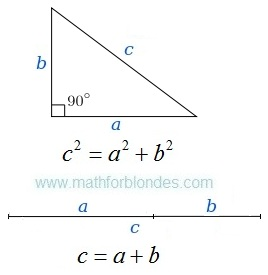Right triangle and two a line segments
How one formula turns into other formula? To see it, we use the law of cosines. We will draw the picture, we will write down conditions, we will execute transformations.Triangle and the law of cosinesRight triangle and the Pythagorean theorem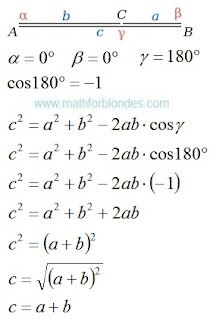The law of cosines and sum of two a line segments
We use the law of cosines and turned the Pythagorean theorem into the sum of two a line segments. Further we will consider an inverse transformation.

If you liked the publication and you want to know more, help me with working on by other publications.

## 2.25.2017

### Law of sines

The law of sines (sine law, sine formula, or sine rule) is an equation relating the lengths of the sides of a triangle (any shape) to the sines of its angles.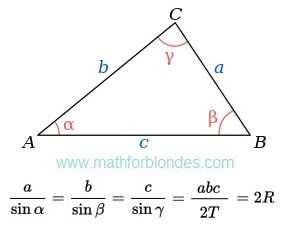The law of sines
Where:
a, b, c - are the lengths of the sides of a triangle;
α, β, γ - are the opposite angles;
T - are the area of triangle;
R - are the radius of the triangle's circumcircle.

How to use this monster? Use dress-making courses. Cut the law of sines on a part.Cut the law of sines on a part
Make a necessary formula of two parts. Use properties of proportions.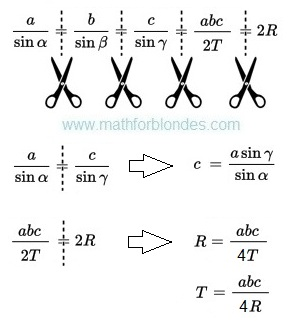Example of use of the law of sine

## 2.13.2017

### Transformations of trigonometric functions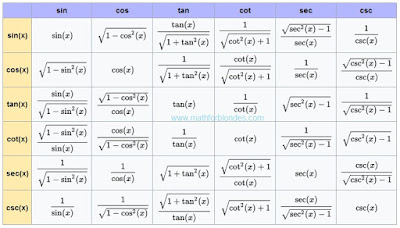Transformations of trigonometric functions
This table shows how one trigonometric functions can be transformed to other trigonometric functions. Sin, cos, tan, cot, sec, csc - all these functions can be transformed.

## 2.10.2017

### Number to the power minus one

What to do if number to the power minus one? Write down this number in a fraction denominator. Here several examples with numbers to the power minus one.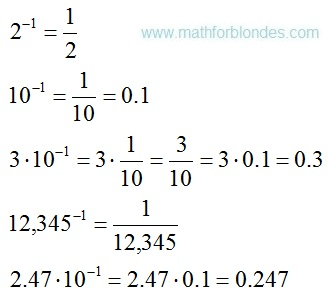Number to the power minus one
Negative exponents of numbers mean that these numbers are in a fraction denominator.Negative exponents

### Trigonometrical circle of a tangent

Last time I drew for you a unit circle of cotangent. Now you can look at a trigonometrical circle of tangent.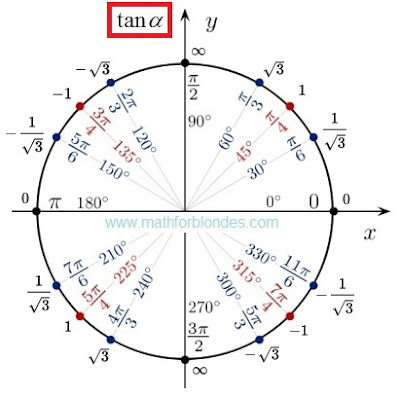Trigonometrical circle of a tangent
There is no sense here. There are angles and values of tangents. If you want to understand sense of a tangent and cotangent, look here.

## 2.08.2017

### Trigonometric tableTrigonometric table are crazy
This trigonometrical table is an example of mathematical marasmus. I am touched by exact values of a sine and cosine. No comments.

If mathematicians do not know what is trigonometric functions, let read here. If mathematicians are not able to divide into zero, let study. I like the idea of this table. I do not like its contents. I corrected this trigonometrical table.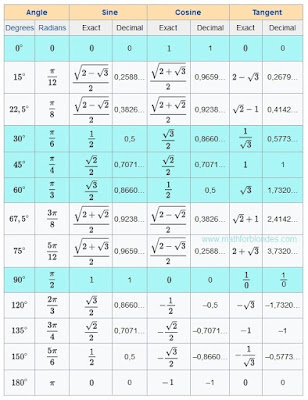Trigonometric table

The most popular angles are highlighted with blue color. 0, 30, 45, 60, 90 degrees most often occur in textbooks.Common fractions will be useful to pupils to fight against teachers. Decimal fractions will be useful physics and to engineers to calculations.

In this trigonometrical table there are no cotangents (cot, cotan, cotg, ctg, ctn). Anything terrible. There are useful formulas which will help you.Useful formulas

Sin 0, 15, 22.5, 30, 45, 60, 67.5, 75, 90, 120, 135, 150, 180 degrees in this table.
Cos 0, Pi/12, pi/8, pi/6, pi/4, pi/3, 3/8 pi, 5/12 pi, pi/2, 2/3 pi, 3/4 pi, 5/6 pi, 1 pi radians.
Tan pi/2 radians or 90 degrees it makes sense.